The innovative discovery in 1831 from Michael Fraday is called the electromagnetic induction. Based on this we do have huge power plants which produce gigawatt hours of electrical energy.

1 The discovery of inductionMoving charges have a magnetic field which influence other moving charges. So current is always combined with a magnetic field. Michael Faraday wanted to know if there is a reverse correlation and observed surprisingly that the existence of a magnetic field will not induce a voltage. But the changing of a magnetic field induced a voltage and if the circuit was closed, a current flew.

This innovative discovery in 1831 from Michael Fraday is called the electro­magnetic induction. It took him nine years to find out. Based on this we do have huge power plants which produce gigawatt hours of electrical energy.

2 Lenz's Law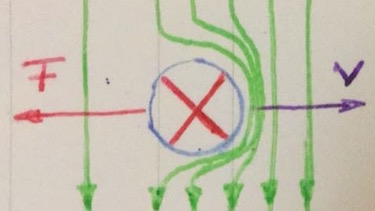If we move a wire with length $l$ through a magnetic field, electrons experience a Lorentz force. Subsequently charges are seperated and a voltage is induced. If we move the wire with constant velocity $v$, the electric and Lorentz force are equal.
\begin{align*} F_{el} &= -F_{L}\\ e \cdot V_{ind}/l &= -e \cdot v \cdot B\\ V_{ind} &= -l \cdot v \cdot B\\ \end{align*}

The magnetic field lines are compressed in the moving direction. With the right hand rule we can determine the direction of current. Lenz's Law: The direction of induced voltage is such, that it will try to oppose the change in flux that is producing it.

3 Lenz's law for a coil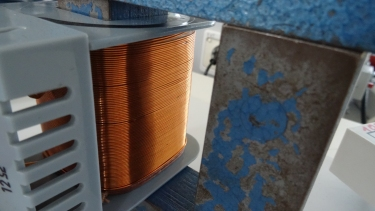If we move a wire loop with the length $l$ through a magnetic field with constant velocity $v$ we can write:

$$V_{ind}= -l \cdot v \cdot B = -B \cdot l \frac{\Delta x}{\Delta t}$$ $$= -B \cdot\frac{\Delta A}{\Delta t}$$

Taking a coil the induced voltage of $N$ loops will be added:

$$V_{ind}=-N \cdot B \cdot \frac{\Delta A}{\Delta t}$$
4 self inductionWhen switching an electric on or off circuit with a coil and resistor, the magnetic field in the coil changes. So a voltage is induced by itself. When switching on, the voltage direction is oppo­site to the applied voltage so current flow will be blocked. When switch­ing off, the voltage direction is similar, in order to keep the current flowing.

$U_L=-U_{ind}=NB\frac{dA}{dt}=$ $\mu_r \mu_0 \frac{N^2A}{l}\frac{dI}{dt}=L\frac{dI}{dt}$

We can compare the coil with an inert millstone. Once running, even if you stop the water, the millstone will still keep turning.

5 switching onSwitching on an electric circuit with a coil and resistor, will result in an exponential function for voltage and current.

$$V_L(t)=V_0 e^{-\frac{t}{\tau}}$$ $$I_L(t)=\frac{V_0}{R} (1-e^{-\frac{t}{\tau}})$$

The time constant $\tau=\frac{L}{R}$ is the elapsed time until the voltage/current has changed to 63 % of its initial value.

6 switching off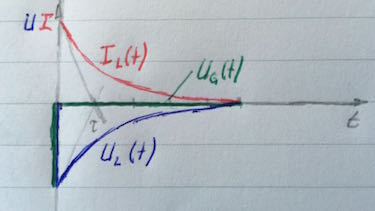Switching off an electric circuit with a coil and resistor, will also result in an exponential function for voltage and current.

$$V_L(t)=-V_0e^{-\frac{t}{\tau}}$$ $$I_L(t)=\frac{V_0}{R}e^{-\frac{t}{\tau}}$$

Self induction is for instance used to switch on a neon tube, since you need a high voltage to ionize the gas inside the tube.

7 induction heating

The alternating magnetic field gets trapped in the base of the pane and induces a voltage. The resulting current heats the pan.
Can you explain why the ceramic surface stays relatively cool?

ideas of
G. Vogt, Elektronikschule Tettnang
J. Grehn, J. Krause, "Metzler-Physik", Schroedel Verlag, 2002
K. Johnson et al., "Advanced Physics for You", Oxfor University Press, 2015

#### Question 1 electric power supplyDuring lunchtime the power counsumption increases. Therefore the electrical water power plant has to increase its electrical energy.

1. Which electrical value increases during lunchtime?
2. Which physical value increases subsequently in the power plant?
3. How can the electrical power plant react in order to avoid a voltage drop of induced voltage?
4. Why is there still a voltage drop for the consumer even the power plant increases their electrical energy?
1. The current.
2. The Lorentz force, so the velocity of the turbine woud decrease.
3. In order to avoid a decrease of velocity and voltage, the power plant has to increase the amount of water on the turbine.
4. Since there is a higher current flow, the voltage drop along the high-voltage transmission line is increased.

#### Question 2 electromagnetic induction

Which description fits?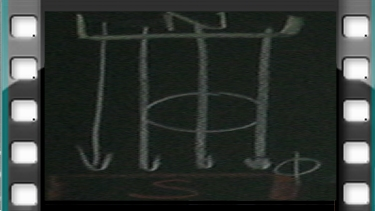1. The wire is moved ... the magnetic field.

2. The wire is moved ...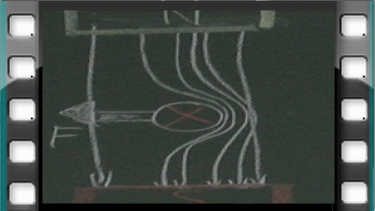1. The wire is moved ...

2. The wire is moved ...

### First relax ...

Here you can find out the dynamo electrical principal, which was invented by Werner von Siemens and made him famous.
Note: This video is only available in German.

#### Question 3 kahoot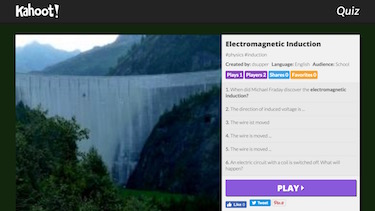Make learning awesome. Play against your colleagues.

#### Question 4 self inductionA RL-circuit is supplied with the following control voltage. Plot the signal for voltage and current,

1. when the RL time constant is 0.4 s,
2. when the RL time constant is 2 s,
3. when the RL time constant is 4 s.
1. when the RL time constant is 0.4 s,2. when the RL time constant is 2 s,3. when the RL time constant is 4 s.